# Height Map System Gear Height Map Height Map

• Slides: 19Height Map System Gear 정찬호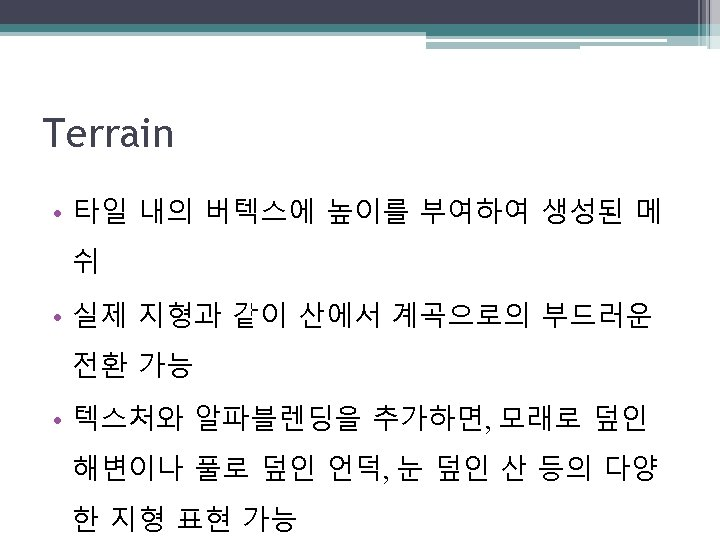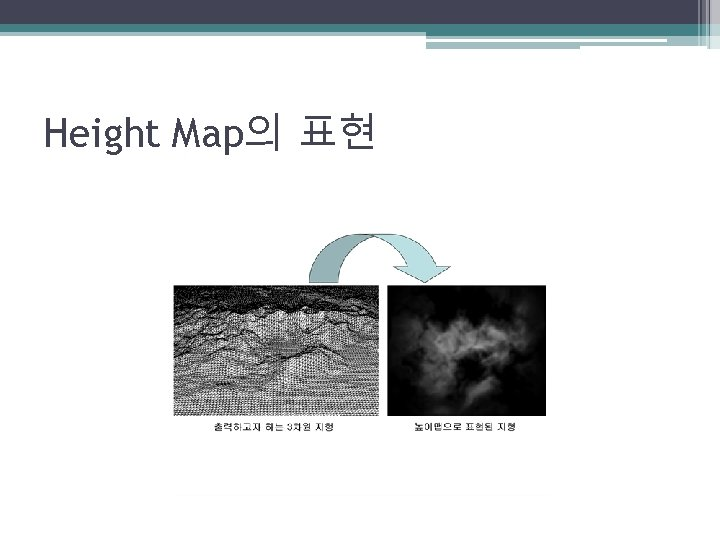Height Map의 표현Height Map의 표현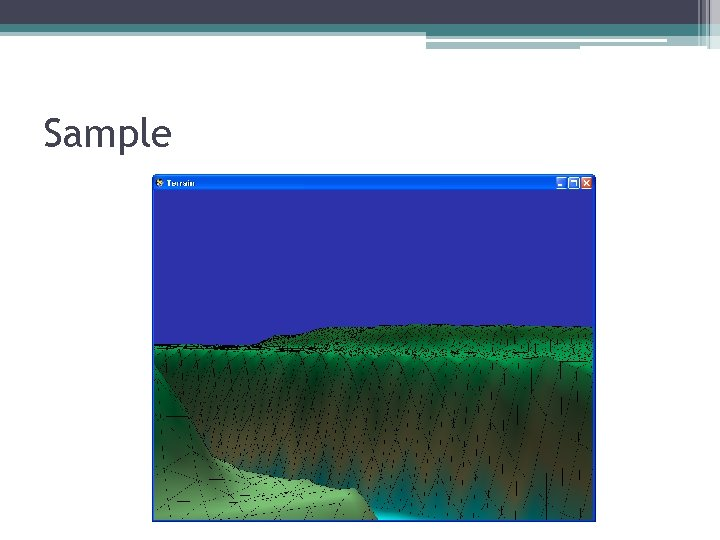SampleHeight map의 생성 원리 Start=(−w/2, d/2) +Z Cell. Spacing Cell/Quad d=Depth +X End=(w/2, −d/2) w=WidthHeight map 생성 과정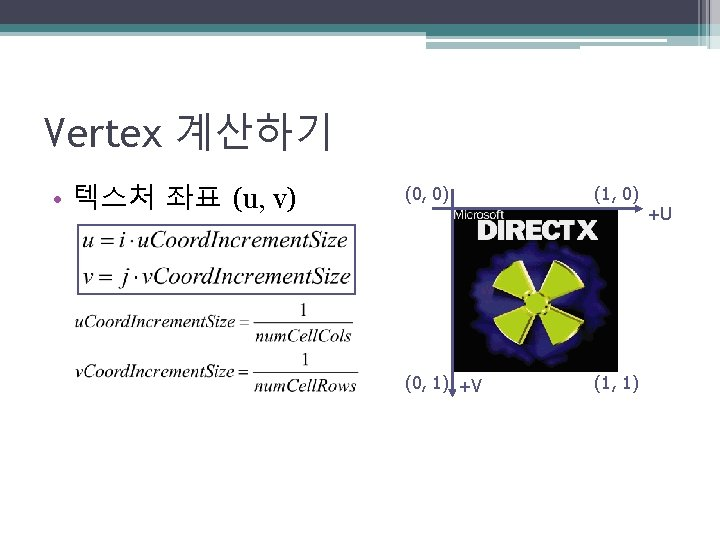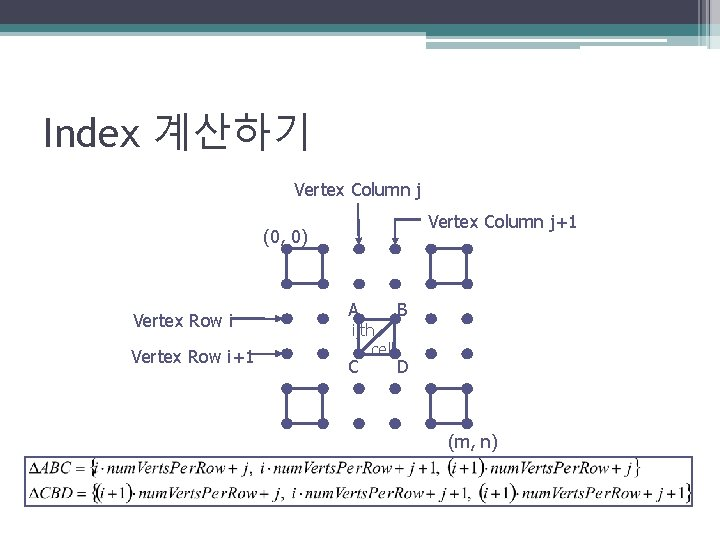Index 계산하기 Vertex Column j+1 (0, 0) Vertex Row i+1 A ijth cell C B D (m, n)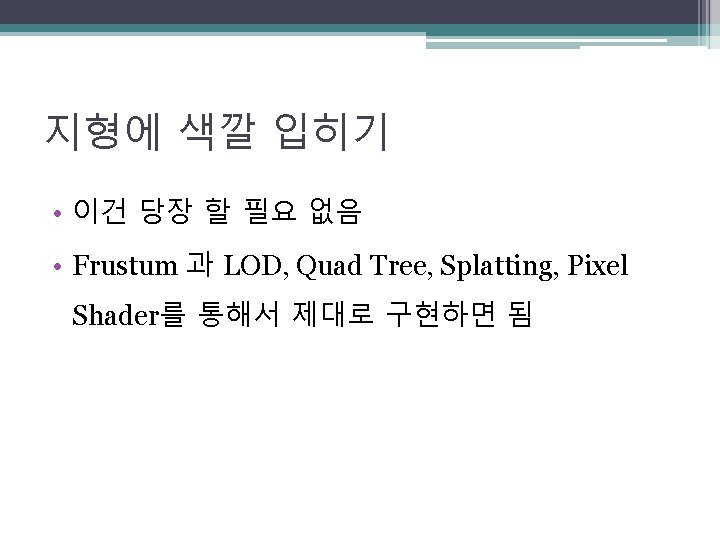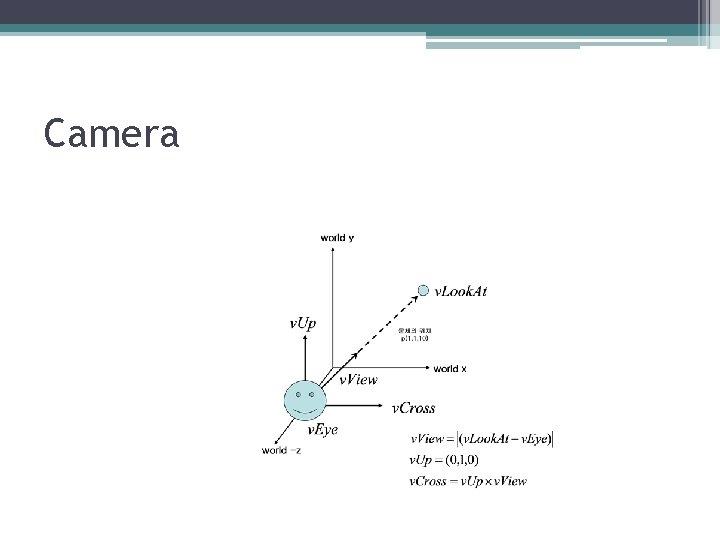Camera지형 위를 걷기 +Z Start Cell. Spacing 1. 0 +X +X p p +Z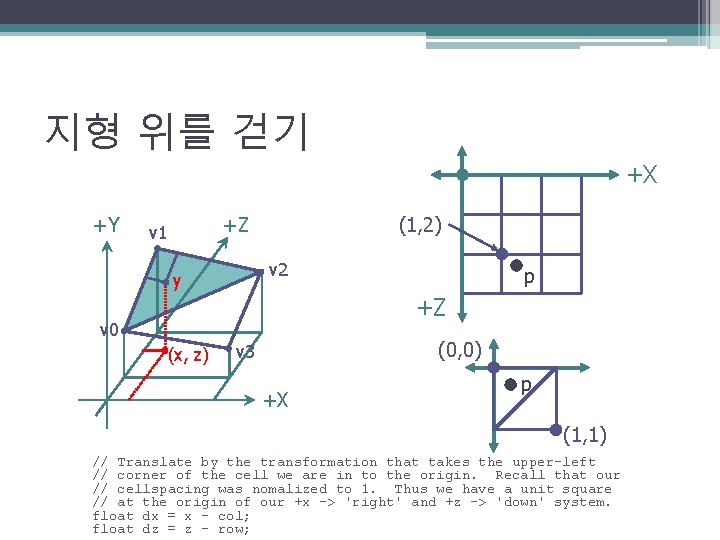지형 위를 걷기 +X +Y (1, 2) +Z v 1 v 2 y p +Z v 0 (x, z) (0, 0) v 3 +X p (1, 1) // Translate by the transformation that takes the upper-left // corner of the cell we are in to the origin. Recall that our // cellspacing was nomalized to 1. Thus we have a unit square // at the origin of our +x -> 'right' and +z -> 'down' system. float dx = x - col; float dz = z - row;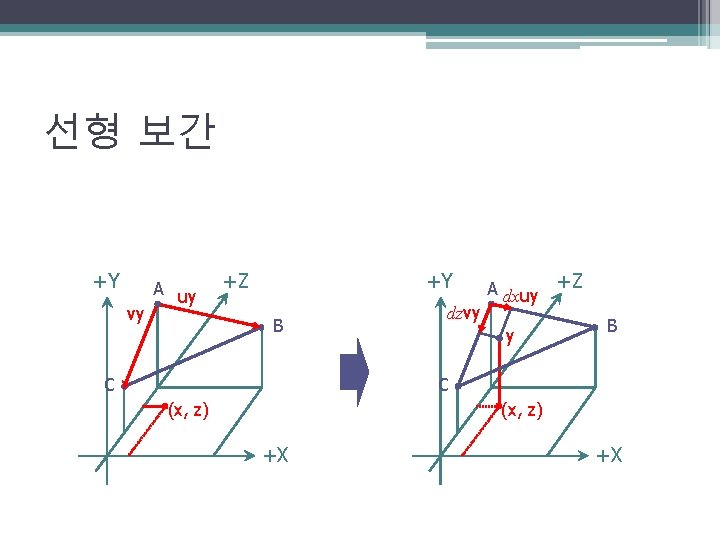선형 보간 +Y vy A uy +Z +Y B C dzvy A dxuy y +Z B C (x, z) +X +X선형 보간 // Note the below computations of u and v are unnecessary, we really // only need the height, but we compute the entire vector to emphasis // the books discussion. float height = 0. 0 f; if(dz < 1. 0 f - dx) // upper triangle ABC { float uy = B - A; // A->B float vy = C - A; // A->C // Linearly interpolate on each vector. The height is the vertex // height the vectors u and v originate from {A}, plus the heights // found by interpolating on each vector u and v. height = A + d 3 d: : Lerp(0. 0 f, uy, dx) + d 3 d: : Lerp(0. 0 f, vy, dz); } else // lower triangle DCB { float uy = C - D; // D->C float vy = B - D; // D->B } // Linearly interpolate on each vector. The height is the vertex // height the vectors u and v originate from {D}, plus the heights // found by interpolating on each vector u and v. height = D + d 3 d: : Lerp(0. 0 f, uy, 1. 0 f-dx) + d 3 d: : Lerp(0. 0 f, vy, 1. 0 f-dz); } float d 3 d: : Lerp(float a, float b, float t) return height; { return a - (a*t) + (b*t); }